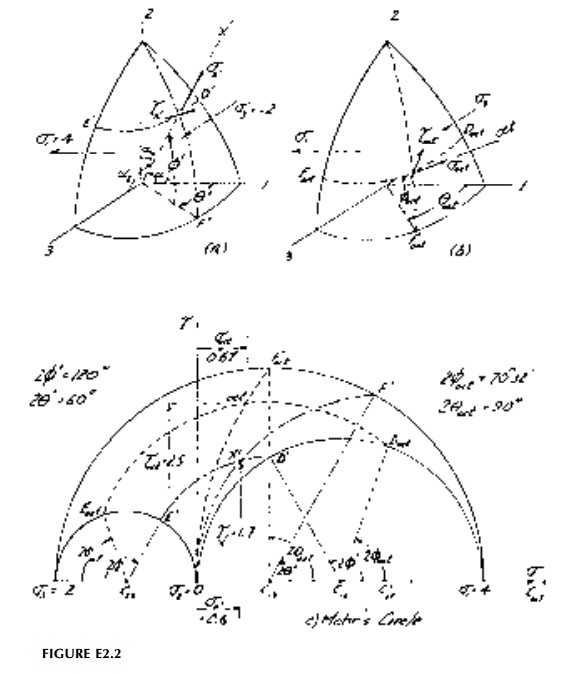Home | | Mechanics of Solids | Mohr's Circle in Three Dimensions

# Mohr's Circle in Three Dimensions

Mohr's circle can be extended to represent three-dimensional symmetric ten-sors such as stress.

Mohr's Circle in Three Dimensions

Mohr's circle can be extended to represent three-dimensional symmetric ten-sors such as stress. Unfortunately it is incomplete in that it can only be con-structed in principal space and even then requires an auxiliary construction (or calculation) to decompose the shear stress resultant. Nevertheless the 3D Mohr's Circle is very useful to portray limiting cases, and particularly the invariants which, as we have seen, appear as stress components (or result-ants) themselves on the octahedral planes.

Referring to Figure 2.10a, once the principal values have been found from the characteristic Equation (2.14), three 2D circles representing each principal planecan be drawn defining the tensor in principal space. Since the principal values are limit values, all other orientations for the tensor must be in the area between these circles (shown slashed).*

There are many ways to then locate a point (say point P) in this principal space, which represents a new orientation of axes. The transformation equations use the direction cosines, but point P can be described more graphically using just two angles: latitude, ?, and longitude, ?, as shown in Figure 2.10a. As we have already seen in 2D, these will be mapped as double angles. Circle BPF of longitude is a 'great circle' (center at 0) so its center must lie along the ? (or E) axis and arc B'F' can be drawn. While circle DPE of latitude is not a great circle, all circles of latitude must have their centers along the same line as the equator AFC at      s1 2 s3 / 2  in stress space. That is, circles of latitude are only seen as circles when looking down the ?2 axis. Thus, again by trial-and-error, arc E'D' can be drawn locating, point P' uniquely in the ? -? (or E-?2) space to determine the normal and shear-resultant components normal and tangential to the new orientation x'. The octahedral orientation along the space diagonal for the same set of stress is shown in Figure 2.10b. Also shown is the maximum shear stress orientation which, in principal space, is actually the quadrahedral state in the 1-3 plane. There are corresponding 1-2 and 2-3 states which are intermediate quadrahedral orientations.

Example 2.2

For the state of stress of Example 2.1 (?1 = 4ksi, ?2 = 0, ?3 = -2ksi), determine using Mohr's Circle (and check by formula)

(a)  the normal and total shear stress on an x' face given by direction cosines l1 = 0.433, m1 = 0.866, n1 = 0.25 and

(b) the octahedral orientation.

(a)Referring to Figure E2.2a:From Equations (2.13)Study Material, Lecturing Notes, Assignment, Reference, Wiki description explanation, brief detail
Civil : Principles of Solid Mechanics : Strain and Stress : Mohr's Circle in Three Dimensions |